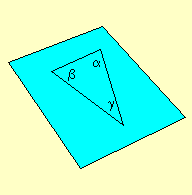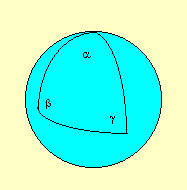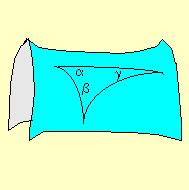Astronomy 162: Professor Barbara Ryden

# CURVATURE OF THE UNIVERSE

``Only two things are infinite, the universe and human stupidity - and I'm not sure about the former.'' - Albert Einstein

## Key Concepts

• On large scales, the universe is apparently homogeneous and isotropic.
• The universe could be positively or negatively curved, but is probably flat.
• The large scale curvature of the universe is determined by its density.

#### (1) On large scales, the universe is apparently homogeneous and isotropic.

First assumption of cosmology: the universe is homogeneous on large scales. ``Homogeneous'' merely means that every region of the universe is pretty much the same as every other region; there are no special locations. On small scales, the universe is obviously very inhomogeneous; it's full of lumps. Stars are much denser than the interstellar medium. Galaxies are much denser than the intergalactic medium. Even superclusters are denser than the voids between them. HOWEVER, on scales larger than superclusters and voids (> 100 Mpc), the universe is finally homogeneous. The average density of stuff within a sphere of radius 100 Mpc is the same as the average density of any other sphere of the same size.

Second assumption of cosmology: the universe is isotropic on large scales. ``Isotropic'' merely means that every direction in the universe is pretty much the same as every other direction; no matter which way you look, you see the same view. On small scales, the universe is obviously anisotropic; there exist preferred directions. Look down & you see rock; look up & you see sky. Look toward the Virgo cluster & you see lots of galaxies; look away from the the Virgo cluster & you see fewer galaxies. HOWEVER, on scales larger than superclusters and voids (> 100 Mpc), the universe is finally isotropic.

Another assumption of cosmology (and of all fields of science) is that the laws of physics are universal; that is, they are the same everywhere in the universe. For instance, we assume that Kepler's Third Law applies to a binary galaxies millions of light years away just as well as it applies to planets within the Solar System.

Combine the assumptions of homogeneity, isotropy, and universality, and you have the cosmological principle. The cosmological principle states that on large scales (> 100 Mpc), the universe looks the same from every vantage point. In particular, we here on Earth are not in a special position in the universe (shades of Copernicus!) The cosmological principle, if true, implies that the universe cannot have an edge or a center. An observer at the edge of the universe would see a very different view from an observer at the center of the universe, thus violating the cosmological principle.

#### (2) The universe could be positively or negatively curved, but is probably flat.

Einstein told us, in his theory of General Relativity, that on small scales, space is ``dimpled'' by massive objects such as stars, galaxies, or clusters of galaxies. On large scales, however, where the assumptions of homogeneity and isotropy apply, space must have the same average curvature everywhere.

Consider the analogy of an ant wandering over the surface of an orange. The ant will encounter small local dimples (the pores of the orange), but if the ant wanders far enough, it will discover that the orange is spherical on average.
On large scales, there are three possibilities for the average curvature of space.

First possibility: Space is FLAT
The two-dimensional analog for flat space is a plane (illustrated below).On a plane, and in flat space, the standard laws of plane geometry apply: for instance, the sum of the vertices of a triangle equals 180 degrees. A plane has infinite area; similarly, flat space has infinite volume.
Second possibility: Space has POSITIVE curvature.
The two-dimensional analog for positively curved space is a sphere, illustrated below.On a sphere, and in positively curved space, the laws of plane geometry no longer apply: the sum of the vertices of a triangle, for instance, is greater than 180 degrees. A sphere has a FINITE area; similarly, positively curved space has FINITE volume (but no edge).
Third possibility: Space has NEGATIVE curvature.
The two-dimensional analog for negatively curved space is a saddle shape (called a hyperboloid by mathematicians), illustrated below.On a hyperboloid, and in negatively curved space, the laws of plane geometry don't apply: the sum of the vertices of a triangle, for instance, is less than 180 degrees. A hyperboloid has an INFINITE area; similarly, a negatively curved space has an INFINITE volume.
So what IS the curvature on large scale? It must be one of the three possibilities, but which?

It's hard to tell, since we see only a limited volume within our cosmic particle horizon. It's comparable to the difficulty that early cultures had in determining that the Earth was spherical -- positively curved -- rather than flat. Actually, it's even worse than you might think, since the local curvature due to stars, galaxies, clusters, and superclusters tends to mask the global positive or negative curvature. (Imagine trying to determine the curvature of the Earth if you were confined to Switzerland. The local curvature, due to the Alps, would totally swamp the global curvature due to the Earth's spherical shape.)

The most promising technique for determining the curvature of the Earth involves looking at the angular size of very distant objects, such as ``hot spots'' in the Cosmic Microwave Background. In flat space, light from the hot spots travels along straight lines. In positively curved space, though, light travels along converging lines. This has the effect of making the hot spots look larger than they would in flat space. Conversely, in a negatively curved space, the hot spots would look smaller than they would in flat space. As it turns out, the actual size of hot spots (about one degree across) is just what cosmologists would have expected in a flat universe. Precise measurements lead cosmologists to conclude that the universe is flat, and thus has infinite volume. (We can't rule out, however, the possibility that the universe has a tiny amount of positive curvature, leading to a universe whose volume is finite, although very very much larger than the volume within our cosmic particle horizon.)

#### (3) The large scale curvature of the universe is determined by its density.

General relativity relates the curvature of space (and of time) to the amount of mass (and energy) in the universe. Space is flat if the density of mass (plus energy divided by c2) is equal to a value known as the critical density. (It's negatively curved if the density is lower, and positively curved if the density is higher.) In an expanding universe like our own, the critical density depends on the expansion rate. The mathematical relations of general relativity reveal that the critical density is proportional to the square of the Hubble constant H0. For the measured value, H0 = 70 km/sec/Mpc, the critical density required for the universe to be perfectly flat turns out to be only 9 x 10-27 kg/meter3. The critical density is equivalent to one hydrogen atom for every 180 liters of volume. This doesn't seem like much, but remember that most of the universe consists of empty intergalactic voids. Dense regions like our galaxy take up a small fraction of the total volume.

The mass of clusters of galaxies can be determined by the application of Kepler's Third Law to galaxies within the cluster. A census of all the clusters of galaxies within a few hundred Mpc of us leads to the conclusion that the mass in clusters only amounts to 30 percent or so of the critical density. What provides the rest of the mass (or energy) required to flatten the universe? The Cosmic Microwave Background, although it has no mass, has energy. Every cubic meter of space contains about 400 million CMB photons. The total energy of all those photons contributes 4 x 10-14 joules per cubic meter. If you divide this energy density by c2 to find an equivalent mass density, it comes to merely 5 x 10-31 kilograms per cubic meter, only 0.005 percent of the critical density. The Cosmic Microwave Background doesn't contribute significantly toward flattening the universe. (And starlight doesn't help, either; all the light emitted by stars during the past 14 billion years has an average energy density less than the Cosmic Microwave Background.)

Where is the rest of the mass (or energy) hidden? [Come back next week for a further discussion of the mysterious hidden energy (or mass)...]

Prof. Barbara Ryden (ryden@astronomy.ohio-state.edu)

Updated: 2003 Mar 6